## Point-Point Distance--2-D

Given two points in the Plane, find the curve which minimizes the distance between them. The Line Element is given by(1)

so the Arc Length between the pointsandis(2)

whereand the quantity we are minimizing is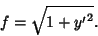(3)

Finding the derivatives gives(4)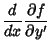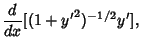(5)

so the Euler-Lagrange Differential Equation becomes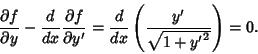(6)

Integrating and rearranging,(7)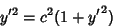(8)(9)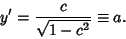(10)

The solution is therefore(11)

which is a straight Line. Now verify that the Arc Length is indeed the straight-line distance between the points.and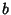are determined from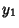(12)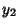(13)

Writing (12) and (13) as a Matrix Equation gives(14)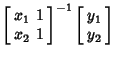(15)

so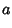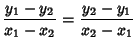(16)(17)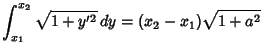(18)

as expected.

The shortest distance between two points on a Sphere is the so-called Great Circle distance.

See also Calculus of Variations, Great Circle, Point-Point Distance--1-D, Point-Point Distance--3-D, Point-Quadratic Distance, Tetrahedron Inscribing, Triangle Inscribing in a Circle

References

Arfken, G. Mathematical Methods for Physicists, 3rd ed. Orlando, FL: Academic Press, pp. 930-931, 1985.